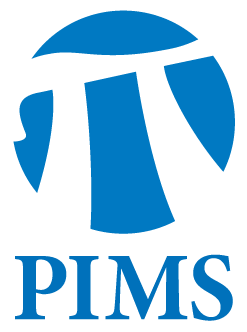## Algebraic Geometry Seminar: Yuri Burda, University of Toronto

• Date: 11/21/2011
Lecturer(s):
Yuri Burda, University of Toronto
Location:

University of British Columbia

Description:

Abstract:

Topological essential dimension of a covering is the
minimal dimension of a base-space such that the original covering can be
induced from some covering over this base-space.

We will see how to compute the topological essential dimension for coverings over tori.

Surprisingly this question turns out to be useful in obtaining
estimates in Klein's resolvent problem: what is the minimal number k
such that the equation z^n+a_1z^n+...+a_n=0 with complex coefficients
a_1,...,a_n can be reduced by means of a rational substitution
y=R(z,a_1,...,a_n) to an equation on y depending on k algebraically
independent parameters.

We will also obtain some bounds in the analogue of this question
for other algebraic functions and get a sharp result for functions on
C^n unramified outside of coordinate hyperplanes.

Schedule:

3:10pm  - 4:10pm in WMAX 110.